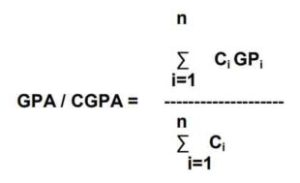# CGPA CALCULATOR SEMESTER 6 REGULATION 2017

## SEMESTER 6 REGULATION 2017 GPA CALCULATOR

### Anna University CGPA Calculator Regulation 2017:

First Calculate the GPA of Each Semester after which you can Calculate the cgpa of semester in below calculator

Semester 1 CGPA Calculator

Semester 2 CGPA Calculator

Semester 3 CGPA Calculator

Semester 4 CGPA Calculator

Semester 5 CGPA Calculator

Semester 6 CGPA Calculator

### Formula Used In CGPA Calculation:

The Formula used to calculate CGPA iswhere

n is number of all courses successfully cleared during the particular semester in the case of GPA

GPi is the point corresponding to the grade obtained for each course

Ci is the number of Credits assigned to the course